Question

# What is the real increase in value if \$2,500 is invested for one year at 7%...

What is the real increase in value if \$2,500 is invested for one year at 7% interest and the rate of inflation during that time is 2.1%

 Nominal rate of interest 7% Inflation rate 2.10% Real rate of return = (1+Nominal rate) /(1+Inflation rate) -1 1.07 /1.021 - 1 =4.80% Amount invested = 2500 Amount after one year = Amount invested (1+reat rate) 2500 *1.048 = 2620 Real increase in value = 2620-2500 =120

#### Earn Coins

Coins can be redeemed for fabulous gifts.

Similar Homework Help Questions
• ### A portfolio manager invested \$1,500,000 in bonds in 2007. In one year the market value of...

A portfolio manager invested \$1,500,000 in bonds in 2007. In one year the market value of the bonds dropped to \$1,485,000. The interest payments during the year totaled \$105,000. a. What was the manager’s total rate of return for the year? The total rate of return is b. What was the manager’s real rate of return if the inflation rate during the year was 2.3%?

• ### You invested \$10,000 one year ago in a stock, and the value of the stock is...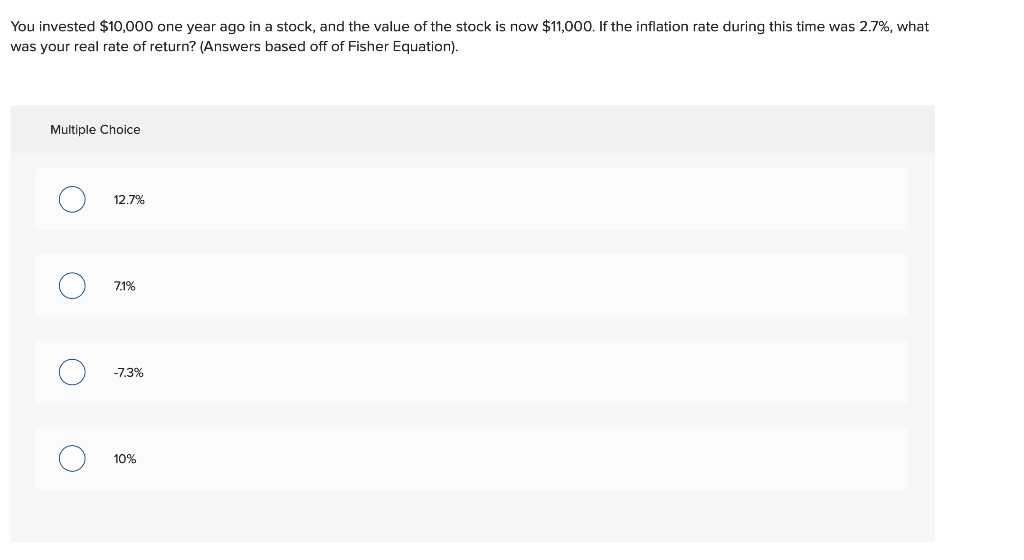You invested \$10,000 one year ago in a stock, and the value of the stock is now \$11,000. If the inflation rate during this time was 2.7%, what was your real rate of return? (Answers based off of Fisher Equation). Multiple Choice O 12.7% O 7.1% O -7.3% O 10%

• ### A portfolio manager invested \$1,400,000 in bonds. In one year, the market value of the bonds...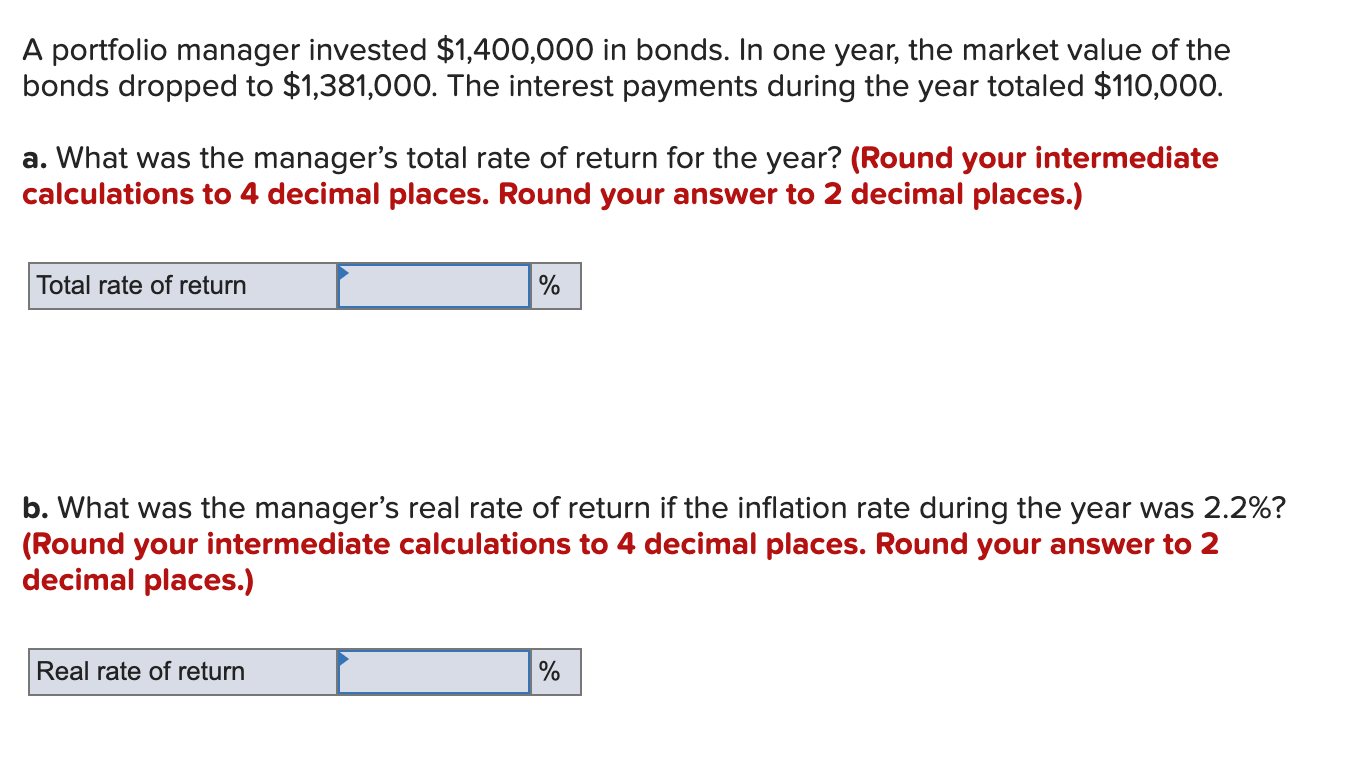A portfolio manager invested \$1,400,000 in bonds. In one year, the market value of the bonds dropped to \$1,381,000. The interest payments during the year totaled \$110,000. a. What was the manager's total rate of return for the year? (Round your intermediate calculations to 4 decimal places. Round your answer to 2 decimal places.) Total rate of return % b. What was the manager's real rate of return if the inflation rate during the year was 2.2%? (Round your intermediate...

• ### A portfolio manager invested \$1,700,000 in bonds. In one year, the market value of the bonds...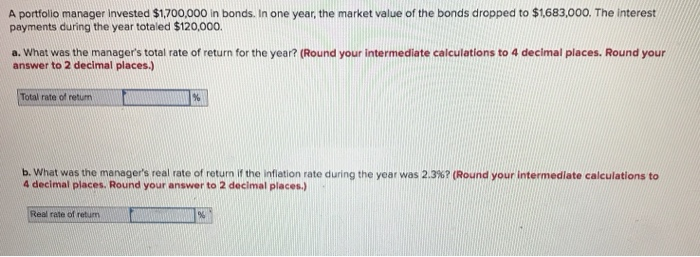A portfolio manager invested \$1,700,000 in bonds. In one year, the market value of the bonds dropped to \$1683,000. The interest payments during the year totaled \$120,000. a. What was the manager's total rate of return for the year? (Round your intermediate calculations to 4 decimal places. Round your answer to 2 decimal places.) Total rate of of return % b. What was the manager's real rate of return if the inflation rate during the year was 2.3%? (Round your...

• ### Re 3 A portfolio manager invested \$2,300,000 in bonds. In one year, the market value of...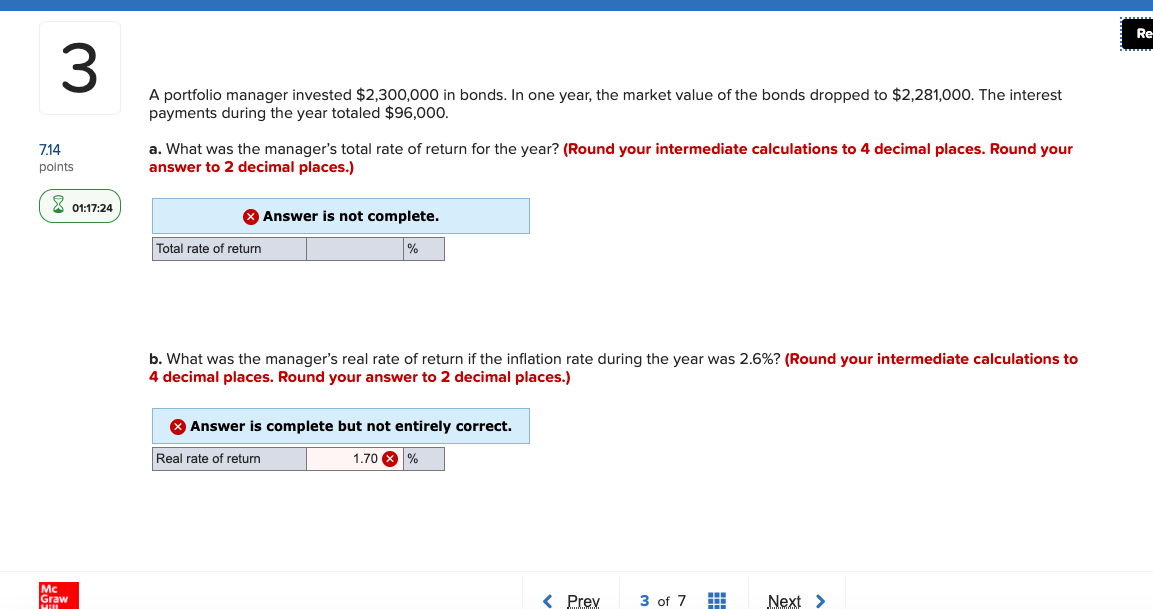Re 3 A portfolio manager invested \$2,300,000 in bonds. In one year, the market value of the bonds dropped to \$2,281,000. The interest payments during the year totaled \$96,000. 7.14 points a. What was the manager's total rate of return for the year? (Round your intermediate calculations to 4 decimal places. Round your answer to 2 decimal places.) 8 01:17:24 Answer is not complete. Total rate of return b. What was the manager's real rate of return if the inflation...

• ### Check my work A portfolio manager invested \$2,300,000 in bonds. In one year, the market value...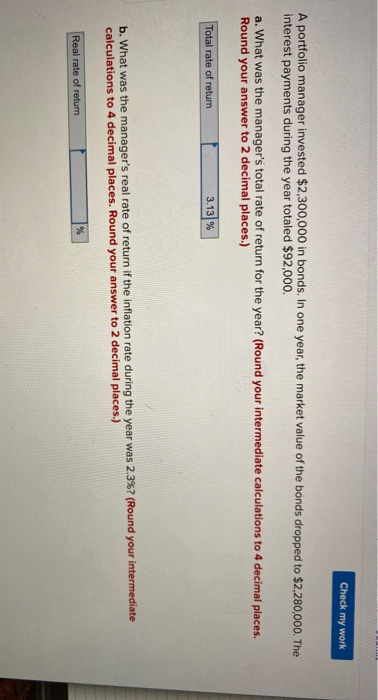Check my work A portfolio manager invested \$2,300,000 in bonds. In one year, the market value of the bonds dropped to \$2,280,000. The interest payments during the year totaled \$92,000. a. What was the manager's total rate of return for the year? (Round your intermediate calculations to 4 decimal places. Round your answer to 2 decimal places.) Total rate of return 3.13% b. What was the manager's real rate of return if the inflation rate during the year was 2.3%?...

• ### If productivity increases from one year to the next, only nominal GDP would increase. only real...

If productivity increases from one year to the next, only nominal GDP would increase. only real GDP would increase. nominal and real gross domestic product would increase. If inflation occurs in a given year, the change in the real measurement (GDP) would be equal to the change in the nominal one. the change in the real measurement (GDP) would be smaller than the change in the nominal one. the change in the real measurement (GDP) would be greater than the...

• ### Jose invested 4,000 a year in two savings accounts with simple interest. Invested 2,500 at 9%...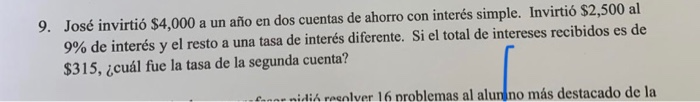Jose invested 4,000 a year in two savings accounts with simple interest. Invested 2,500 at 9% interest and the rest at a different interest rate. If the total interest received is \$ 315. What was the rate of the second account? 9. José invirtió \$4,000 a un año en dos cuentas de ahorro con interés simple. Invirtió \$2,500 al 9% de interés y el resto a una tasa de interés diferente. Si el total de intereses recibidos es de \$315,...

• ### 1. The best definition of inflation is a(n): a temporary increase in prices. b. increase in...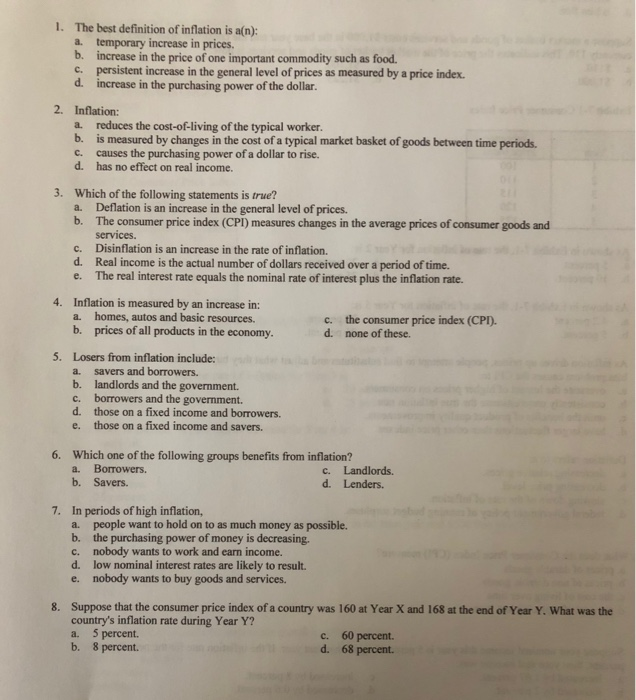1. The best definition of inflation is a(n): a temporary increase in prices. b. increase in the price of one important commodity such as food. c. persistent increase in the general level of prices as measured by a price index. d. increase in the purchasing power of the dollar. 2. Inflation: a. reduces the cost-of-living of the typical worker. b. is measured by changes in the cost of a typical market basket of goods between time periods. c. causes the...

• ### The real risk-free rate of interest is 1.2%. Inflation is expected to be 7% this year...

The real risk-free rate of interest is 1.2%. Inflation is expected to be 7% this year and 6% during the next 2 years. Assume that the maturity risk premiums is zero. What is the yield on 1-year treasury securities? Write your answer as a percent